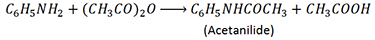MORE IN Applied Chemistry 2
MU First Year Engineering (Semester 2)
Applied Chemistry 2
May 2012
Total marks: --
Total time: --
INSTRUCTIONS
(1) Assume appropriate data and state your reasons
(2) Marks are given to the right of every question
(3) Draw neat diagrams wherever necessary

Solve any five from the following:
1 (a) Give the anode and cathode reactions involved in the corrosion of steel pipe connected to copper plumbing.
3 M
1 (b) Give the composition and uses of Duralumin.
3 M
1 (c) 1g of coal sample was used for determination of Nitrogen by Kjeldhal's method. The ammonia evolved was passed into 50ml of 0.1N sulphuric acid. The excess acid required 42ml of 0.1N NaOH for neutralization. Calculate the percentage of N2.
3 M
1 (d) What are green solvents? Give two industrial applications of green solvents.
3 M
1 (e) Explain the statement A catalyst does not affect the position of equilibrium in a reversible reaction .
3 M
1 (f) Give the classification of composite materials.
3 M

2 (a) Discuss the effect of the following factors on the rate of corrosion:
i. Relative area of anode and cathode.
ii. pH
iii. Over voltage
6 M
2 (b) An electric current is passed through two cells arranged in series containing AgNO3 and ZnSO4 solutions with platinum electrons. If 216mg of silver is deposited in AgNO3/Pt cell, calculate the amount of Zinc deposited in ZnSO4/Pt cell. (At.wt of Ag=108 and Zn=65)
5 M
2 (c) What is knocking? Define octane number. What is unleaded petrol?
4 M

3 (a) Explain conventional and green route of manufacturing Adipic acid. Also justify why the route is green.
6 M
3 (b) What is cathodic protection? Discuss the sacrificial anode method of corrosion control.
5 M
3 (c) A sample of coal has the following composition: C = 90%, H = 8%, N = 2%, S = 1.5%, O = 5% and remaining ash. Calculate the GCV and LCV (Latent heat of condensation of steam = 587cal/g).
4 M

4 (a) What is meant by activation energy? How does catalyst affect activation energy of a reaction? Explain with at least one example the role of metal oxides as a catalyst.
6 M
4 (b) What are composite materials? Describe fibre reinforced composites.
5 M
4 (c) What is Powder metallurgy? Explain cold powder extrusion moulding.
4 M

5 (a) Explain concentration cell corrosion with the help of suitable examples.
6 M
5 (b) A gaseous fuel has following composition by volume: H2 = 35%, CH4 = 45%, C2H6 = 6%, CO = 12% and remaining N2. Calculate the minimum amount of air required 27°C and 760 mm Hg pressure for the complete combustion of 1m3 of the fuel.
5 M
5 (c) What are ceramic powders? Discuss the manufacture and uses of alumina.
4 M

6 (a) Discuss the refining of petroleum. Also give the different fractions with their boiling ranges.
6 M
6 (b) Explain absorption theory of catalysis.
5 M
6 (c) Calculate percentage atom economy for the following reaction with respect to acetanilide:4 M

7 (a) What is cracking? Discuss the fixed bed catalytic cracking method in detail.
6 M
7 (b) Discuss electroplating method of applying metallic coating in detail.
5 M
7 (c) An air dried sample of coal weighing 2.9g was taken for volatile matter determination. After losing volatile matter the coal sample weighed 1.96g. If it contains 4.5% moisture, find the percentage volatile matter in it.
4 M

More question papers from Applied Chemistry 2